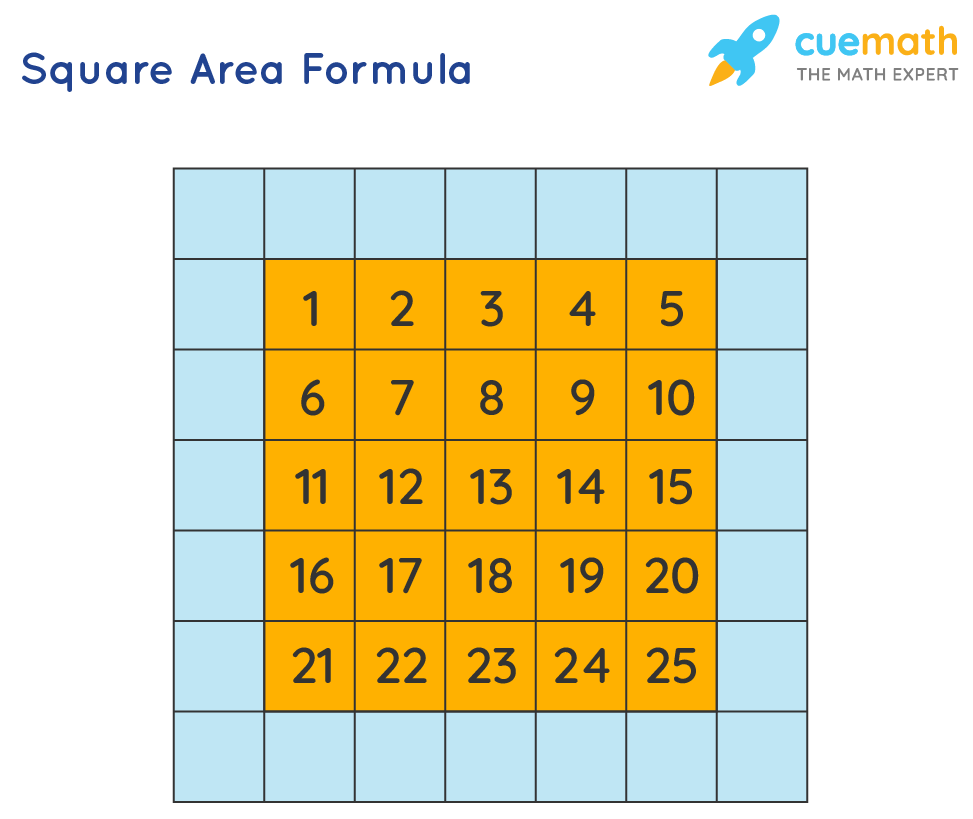# Square Area Formula

The area of a square is the space occupied by the square.

Look at the green square shown below.

It has occupied 25 square units.Therefore, the area of the square is 25 square units.

## What is the Square Area Formula?

The area of a square is the product of its sides.

The formula for the area of a square with side 's' is:
Area of a Square = side x side = side2

When the diagonal d of the square is known, the formula for finding the area of the square is:
Area of a Square = d2/2

## Solved Examples Using Square Area Formula

### What is the area of a square swimming pool of sides 8 ft each?

Solution:

We know, that the area of a square = side2

Therefore, the area of the swimming pool is: 8 x 8 = 64 sq. ft

Answer: The area of the swimming pool is 64 sq. ft

### The area of a square carrom board is 3600 sq. in. What is the length of its sides?

Solution:

Area of a square = side2

Area of the square carrom board = 3600 sq. in.

We know that 60 x 60 gives 3600

### Answer: Side of the carrom board = 60 in.

Learn from the best math teachers and top your exams

• Live one on one classroom and doubt clearing
• Practice worksheets in and after class for conceptual clarity
• Personalized curriculum to keep up with school